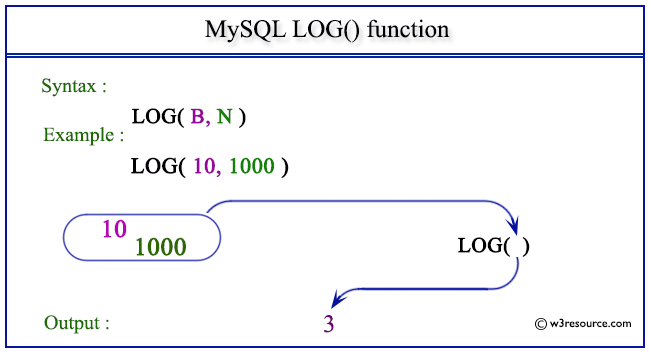# MySQL LOG() function

## LOG() function

MySQL LOG() returns the natural logarithm of a number that is the base e logarithm of the number.

The return value will be NULL when the value of the number is less than or equal to 0.

The EXP() is the inverse of this LOG(). When LOG() execute with two parameters it returns the logarithm of the number to the base specified as a number in the first argument.

The return value will be NULL when the value of the number is less than or equal to 0 or the value of the number specified as the first argument is less than or equal to 1.

Syntax:

```LOG (N), LOG(B, N);
```

Argument

Name Description
N A number.
B Base of the logarithm of N
N A number.

Note: LOG(B,N) is equivalent to LOG(N) / LOG(B).

Pictorial presentation of MySQL LOG() functionExample of MySQL LOG() function

Code:

``````SELECT LOG(3);
```
```

Explanation:

The above MySQL statement returns the natural logarithm of 3.

Sample Output:

```mysql> SELECT LOG(3);
+------------------+
| LOG(3)           |
+------------------+
| 1.09861228866811 |
+------------------+
1 row in set (0.00 sec)
```

Example : LOG() function using negative value

Code:

``````SELECT LOG(-3);
```
```

Explanation:

The above MySQL statement returns NULL because the number specified in the argument is less than 0.

Sample Output:

```mysql> SELECT LOG(-3);
+---------+
| LOG(-3) |
+---------+
|    NULL |
+---------+
1 row in set (0.00 sec)
```

Example: LOG() function using base of a number system

Code:

``````SELECT LOG(10,1000);
```
```

Explanation:

The above MySQL statement returns the natural logarithm of 1000 divided by the natural logarithm of 10.

Sample Output:

```mysql> SELECT LOG(10,1000);
+--------------+
| LOG(10,1000) |
+--------------+
|            3 |
+--------------+
1 row in set (0.00 sec)
```

Example: LOG() with a smaller 1st argument

Code:

``````SELECT LOG(1,1000);
```
```

Explanation:

The above MySQL statement returns NULL because the value of the first number is 1.

Sample Output:

```mysql> SELECT LOG(1,1000);
+-------------+
| LOG(1,1000) |
+-------------+
|        NULL |
+-------------+
1 row in set (0.00 sec)
```

All Mathematical FunctionsPrevious: LN()
Next: LOG2()

﻿